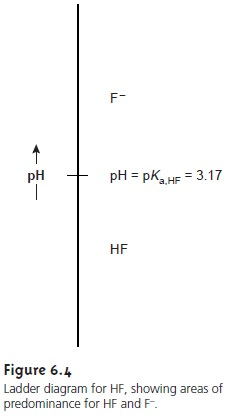Home | | Modern Analytical Chemistry | Ladder Diagrams for Acidâ€“Base Equilibria

# Ladder Diagrams for Acidâ€“Base Equilibria

To see how a ladder diagram is constructed, we will use the acidâ€“base equilibrium between HF and Fâ€“

To see how a ladder diagram is constructed, we will use the acidâ€“base equilibrium between HF and Fâ€“Finally, replacing the negative log terms with p-functions and rearranging leaves us withExamining equation 6.31 tells us a great deal about the relationship between pH and the relative amounts of Fâ€“ and HF at equilibrium. If the concentrations of Fâ€“ and HF are equal, then equation 6.31 reduces to

pH= pKa,HF = â€“log(Ka,HF) = â€“log(6.8 x 10â€“4) = 3.17

For concentrations of Fâ€“ greater than that of HF, the log term in equation 6.31 is positive and

pH> pKa,HF or pH > 3.17

This is a reasonable result since we expect the concentration of hydrofluoric acidâ€™s conjugate base, Fâ€“, to increase as the pH increases. Similar reasoning shows that the concentration of HF exceeds that of Fâ€“ when

pH < pKa,HF or pH < 3.17

Now we are ready to construct the ladder diagram for HF (Figure 6.4). The ladder diagram consists of a vertical scale of pH values oriented so that smaller (more acidic) pH levels are at the bottom and larger (more basic) pH levels are at the top. A horizontal line is drawn at a pH equal to pKa,HF. This line, or step, separates the solution into regions where each of the two conjugate forms of HF predominate.By referring to the ladder diagram, we see that at a pH of 2.5 hydrofluoric acid will exist predominately as HF. If we add sufficient base to the solution such that the pH increases to 4.5, the predominate form be- comes Fâ€“.

Figure 6.5 shows a second ladder diagram containing information about  HF/Fâ€“ and NH4 +/NH3 .  From this ladder diagram we see that if the pH is less than 3.17, the predominate species are HF and NH4+. For pHâ€™s between 3.17 and 9.24 the predominate species are Fâ€“ and NH4+, whereas above a pH of 9.24 the predominate species are Fâ€“ and NH3.Ladder diagrams are particularly useful for evaluating the reactivity of acids and bases. An acid and a base cannot coexist if their respective areas of predominance do not overlap. If we mix together solutions of NH3 and HF, the reactionoccurs because the predominance areas for HF and NH3 do not overlap. Be- fore continuing, let us show that this conclusion is reasonable by calculating the equilibrium constant for reaction 6.32. To do so we need the following three reactions and their equilibrium constants.Since the equilibrium constant is significantly greater than 1, the reactionâ€™s equilib- rium position lies far to the right. This conclusion is general and applies to all lad- der diagrams. The following example shows how we can use the ladder diagram in Figure 6.5 to evaluate the composition of any solution prepared by mixing together solutions of HF and NH3.If the areas of predominance for an acid and a base overlap each other, then practically no reaction occurs. For example, if we mix together solutions of NaF and NH4Cl, we expect that there will be no significant change in the moles of Fâ€“ and NH4+. Furthermore, the pH of the mixture must be between 3.17 and 9.24. Because Fâ€“ and NH4+ can coexist over a range of pHs we cannot be more specific in estimat- ing the solutionâ€™s pH.

The ladder diagram for HF/Fâ€“ also can be used to evaluate the effect of pH on other equilibria that include either HF or Fâ€“. For example, the solubility of CaF2

CaF2(s)  < = = = >  tCa2+(aq)+ 2Fâ€“(aq)

is affected by pH because Fâ€“ is a weak base. Using Le ChĂ˘telierâ€™s principle, if Fâ€“ is converted to HF, the solubility of CaF2 will increase. To minimize the solubility of CaF2 we want to control the solutionâ€™s pH so that Fâ€“ is the predominate species. From the ladder diagram we see that maintaining a pH of more than 3.17 ensures that solubility losses are minimal.

Study Material, Lecturing Notes, Assignment, Reference, Wiki description explanation, brief detail
Modern Analytical Chemistry: Equilibrium Chemistry : Ladder Diagrams for Acidâ€“Base Equilibria |